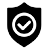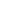#### WhatsApp Quick Chat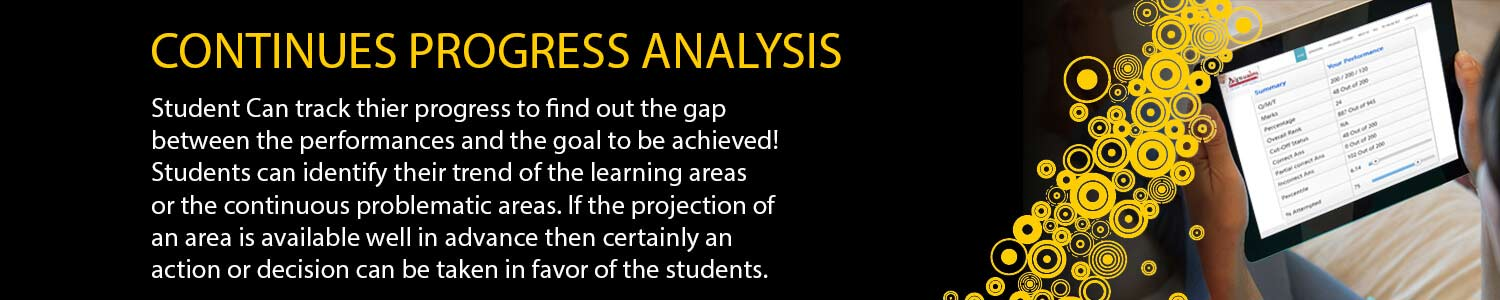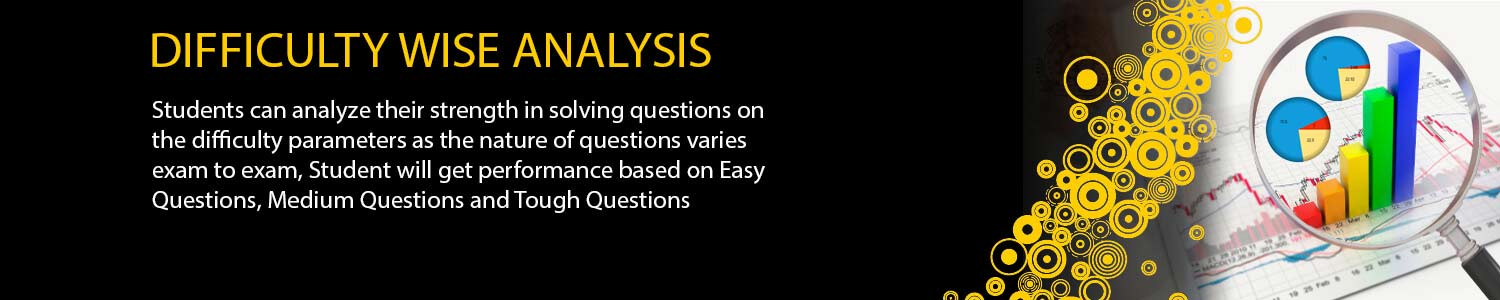## IIT JAM MATH

#### Offers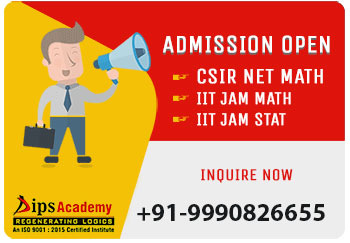##### News & Events# Correspondences courses for IIT JAM Maths

## JAM Maths Online Test Series

If you want to crack any competitive examination, then not only the understanding of the concept, but also practicing of the numerous numbers of questions relative to those concepts is necessary. You can divide it into two parts among them one part is preparing well for Exam and implementation of that knowledge and shortcuts on the exam day is another part. A large number of students become unsuccessful due to the lack of exam temperament and adoption of wrong approach on the exam day.

To overcome these obstacles students should have joined the test series, which gives them proper exam temperament and guide them according to their performance in these mock tests so that students become habitual of these kind of temperament and do not caught by nervousness at the time of exam.

Our test is designed in such a way so that you can get a large number of standard questions on every minute concept and provides proper exam temperament. This student becomes habitual to solve that number of questions in the given time and since our format of exam is same as per the exam conducting body so it helps them to perform well on the exam day.

##### Main features

Quality of the question as per the norms of Exam conducting body.

Question papers are designed as per latest exam's pattern.

You can get question on each and every concept which helps them in understanding the whole concept thoroughly.

Freedom to avail the test Topic/Concept wise and full length

Your whole performance is analyzed and displayed to you

Get Answer and solution post test

Question Papers are true copy of actual exam pattern.

Can give the test without leaving the comfort of your home#### JAM All India Ranker

(Online Test Series)

12 Tests (8 Module-wise & 4 Full Length).#### JAM Real Time

(Online Test Series)

8 Full Length Test (4 New + 4 Old).#### JAM Anytime

(Online Test JAM)

26 Test (12 New + 14 Old)

### IIT-JAM MATH TEST SERIES SCHEDULE 2024 (ONLINE)

TEST TYPE Modules DATE Syllabus
MWT-01 Real Analysis: I 02-Jan-24 Sequences and Series of Real Numbers: convergence of sequences, bounded and monotone sequences, Cauchy sequences, Bolzano-Weierstrass theorem, absolute convergence, tests of convergence for series – comparison test, ratio test, root test; Power series (of one real variable), radius and interval of convergence, term-wise differentiation and integration of power series. : limit, continuity, intermediate value property, differentiation, Rolle’s Theorem, mean value theorem, L'Hospital rule,
MWT-02 Real Analysis: II 05-Jan-24 Taylor's theorem, Taylor’s series, maxima and minima, Riemann integration (definite integrals and their properties), fundamental theorem of calculus. Functions of Two or Three Real Variables: limit, continuity, partial derivatives, total derivative, maxima and minima. radius and interval of convergence, term-wise differentiation and integration of power series. Multivariable Calculus & Differential Equations: Functions of Two or Three Real Variabels: Limit, continuity, partial derivatives, maxima and minma.
MWT-03 Group Theory 08-Jan-24 Groups: cyclic groups, abelian groups, non-abelian groups, permutation groups, normal subgroups, quotient groups, Lagranges theorem for finite groups, group homomorphisms.
MWT-04 Linear Algebra I 11-Jan-24 Finite Dimensional Vector Spaces: linear independence of vectors, basis, dimension, linear transformations, matrix representation, range space, null space, rank-nullity theorem.
MWT-05 Linear Algebra II 14-Jan-24 Matrices: systems of linear equations, rank, nullity, rank-nullity theorem, inverse, determinant, eigenvalues, eigenvectors.
MWT-06 Group Theory + Linear Algebra 17-Jan-24 Matrices: systems of linear equations, rank, nullity, rank-nullity theorem, inverse, determinant, eigenvalues, eigenvectors. Finite Dimensional Vector Spaces: linear independence of vectors, basis, dimension, linear transformations, matrix representation, range space, null space, rank-nullity theorem. Groups: cyclic groups, abelian groups, non-abelian groups, permutation groups, normal subgroups, quotient groups, Lagranges theorem for finite groups, group homomorphisms.
MWT-07 Integral Calculus + ODE 20-Jan-24 Integral Calculus: double and triple integrals, change of order of integration, calculating surface areas and volumes using double integrals, calculating volumes using triple integrals. Differential Equations: Bernoulli’s equation, exact differential equations, integrating factors, orthogonal trajectories, homogeneous differential equations, method of separation of variables, linear differential equations of second order with constant coefficients, method of variation of parameters, Cauchy-Euler equation.
MWT-08 Real Analysis 23-Jan-24 Sequences and Series of Real Numbers: convergence of sequences, bounded and monotone sequences, Cauchy sequences, Bolzano-Weierstrass theorem, absolute convergence, tests of convergence for series – comparison test, ratio test, root test; Power series (of one real variable), radius and interval of convergence, term-wise differentiation and integration of power series. Functions of One Real Variable: limit, continuity, intermediate value property, differentiation, Rolle’s Theorem, mean value theorem, L'Hospital rule, Taylor's theorem, Taylor’s series, maxima and minima, Riemann integration (definite integrals and their properties), fundamental theorem of calculus. Functions of Two or Three Real Variables: limit, continuity, partial derivatives, total derivative, maxima and minima.
FLT-01 Full Length Test 27-Jan-24 As per Exam Pattern
FLT-02 Full Length Test 30-Jan-24 As per Exam Pattern
FLT-03 Full Length Test 03-Feb-24 As per Exam Pattern
FLT-04 Full Length Test 06-Feb-24 As per Exam Pattern
• > Total Questions (Each Module Wise Test): 40

• > Total Marks (Each Module Wise Test): 65

• > Total Duration (Each Module Wise Test): 120 Minutes

## Offers## News & Events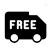##### Free Delivery